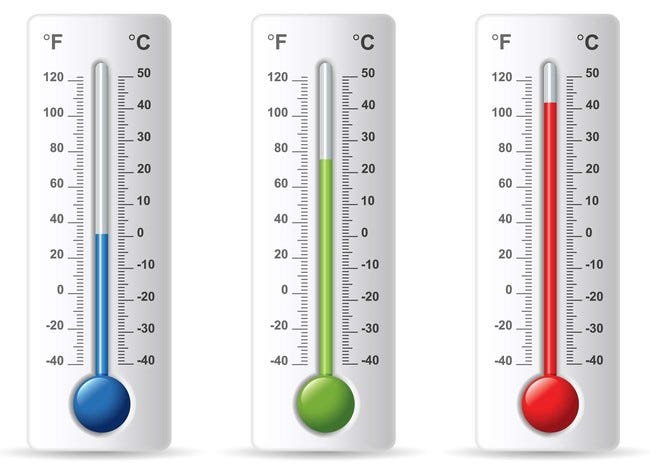# Temperature Degrees Converter: Celsius to Fahrenheit and Fahrenheit to Celsius

0
24Temperature is an important aspect of our daily lives, whether we are trying to stay cool during the hot summer months or keeping warm during the cold winter season. Different parts of the world use different temperature units, which can sometimes be confusing when trying to understand or compare temperatures. The most commonly used temperature units are Celsius and Fahrenheit, and converting between these units is essential for accurate temperature readings. This is where a temperature degrees converter comes in handy.

Celsius and Fahrenheit are two different temperature scales, and each has its own advantages and disadvantages. Celsius is based on the metric system and is widely used around the world, especially in scientific applications. Fahrenheit, on the other hand, is used primarily in the United States and some other countries, but is slowly being phased out in favor of Celsius. Regardless of the scale used, it is important to be able to convert between Celsius and Fahrenheit to accurately interpret temperature measurements.

To convert from Celsius to Fahrenheit, you can use the formula:

F = (C x 1.8) + 32

Where F is the temperature in Fahrenheit and C is the temperature in Celsius. For example, if you want to convert 20 degrees Celsius to Fahrenheit, you would use the formula:

F = (20 x 1.8) + 32 = 68 degrees Fahrenheit

Conversely, to convert from Fahrenheit to Celsius, you can use the formula:

C = (F – 32) / 1.8

For example, if you want to convert 68 degrees Fahrenheit to Celsius, you would use the formula:

C = (68 – 32) / 1.8 = 20 degrees Celsius

While it is possible to manually calculate these conversions, it can be time-consuming and prone to errors. This is where a temperature degrees converter comes in handy. With a temperature degrees converter, you can quickly and easily convert between Celsius and Fahrenheit with just a few clicks.

There are many temperature degrees converters available online, and most are free to use. Simply input the temperature value in the desired scale, and the converter will automatically calculate the equivalent value in the other scale. Some temperature degrees converters may also provide additional features, such as the ability to convert between Kelvin and other temperature units.

In addition to online temperature degrees converters, many electronic devices such as smartphones and digital thermometers also have built-in converters. This makes it easy to obtain accurate temperature readings in either Celsius or Fahrenheit, depending on your preference or location.

It is important to note that while temperature degrees converters can provide accurate conversions, they do not account for factors such as humidity or altitude, which can affect the perceived temperature. For example, a temperature of 20 °C in a humid environment may feel warmer than the same temperature in a dry environment. Additionally, altitude can affect the boiling point of water and thus the temperature at which food is cooked.